# Electronics and Communication Engineering - Signals and Systems

Exercise : Signals and Systems - Section 5
1.
The unit step response of a system starting from rest is given by c(t) = 1 - e-2t for t ≥ 0. The transfer function of the syste is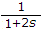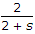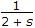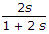Explanation:
No answer description is available. Let's discuss.

2.
check the following system for causality
1. y[n] = x[n] + 2x[n - 1]
2. y[n] =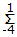x[n - k]
3. y[n] = x[2n]
(1) and (2)
(2) and (3)
(1) omly
(1) and (3)
Explanation:
No answer description is available. Let's discuss.

3.
If the system transfer function of a discrete time system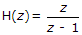then system is
stable
unstable
stable at z = 1
unstable at z = 1
Explanation:
No answer description is available. Let's discuss.

4.
Two rectangular waveforms of duration t1 and t2 seconds are convolved. What is the shape of the resulting waveform?
Triangular
Rectangular
Trapezoidal
Semi-circular